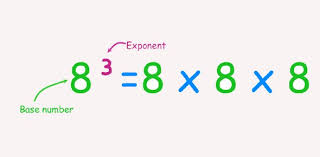# Java Program To Calculate Exponent Value | 4 Ways

Java program to calculate exponent – In this article, we will brief in on all the ways to calculate the exponent in Java programming.

Suitable examples and sample programs have been added to the article for better understanding. The compiler has also been added so that you can execute the programs easily.

The exponent of a number is the number of times that particular number is multiplied with itself.As you can see in the image uploaded above, an exponent is placed at the superscript position of a number which is known as the base.

In the example, 83 is given where 8 is the base and 3 is the exponent.

So, the number 8 is to be multiplied thrice.

83 = 8 * 8 * 8 = 512

Thus, the multiple ways to calculate the exponent in Java programming are as follows:

1.

Output:

2.

Output:

3.

Output:
4.
Output:
5.
Output:
x

## Java Program to Add Two Matrices – 4 Ways | Programs

Java program to add two matrices – The following Java Code will let you know ...